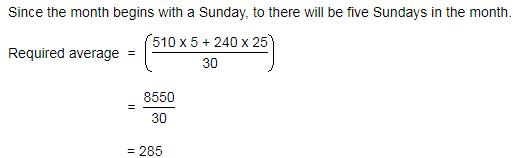Courses

# Test: Average- 1

## 10 Questions MCQ Test IBPS PO Mains - Study Material, Online Tests, Previous Year | Test: Average- 1

Description
This mock test of Test: Average- 1 for Quant helps you for every Quant entrance exam. This contains 10 Multiple Choice Questions for Quant Test: Average- 1 (mcq) to study with solutions a complete question bank. The solved questions answers in this Test: Average- 1 quiz give you a good mix of easy questions and tough questions. Quant students definitely take this Test: Average- 1 exercise for a better result in the exam. You can find other Test: Average- 1 extra questions, long questions & short questions for Quant on EduRev as well by searching above.
QUESTION: 1

### A car owner buys diesel at Rs.7.50, Rs. 8 and Rs. 8.50 per litre for three successive years. What approximately is the average cost per litre of diesel if he spends Rs. 4000 each year?

Solution: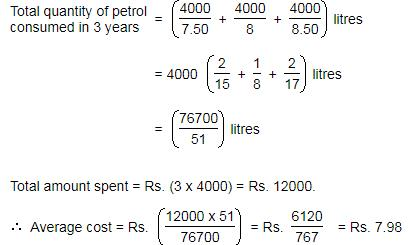QUESTION: 2

### In the first 10 overs of a cricket game, the run rate was only 3.2. What should be the run rate in the remaining 40 overs to reach the target of 282 runs?

Solution:

In 10 overs, run rate was 3.2

Score = run rate x overs

Score = 3.2 x 10

= 32

Target = 282

Score required in 40 Overs = 282 - 32

= 250

Hence, run rate in the remaining 40 overs = 250/40

= 6.25

OR..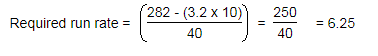QUESTION: 3

### In Arun's opinion, his weight is greater than 65 kg but less than 72 kg. His brother does not agree with Arun and he thinks that Arun's weight is greater than 60 kg but less than 70 kg. His mother's view is that his weight cannot be greater than 68 kg. If all are them are correct in their estimation, what is the average of different probable weights of Arun?

Solution:

Let Arun's weight by X kg.

According to Arun, 65 < X < 72

According to Arun's brother, 60 < X < 70.

According to Arun's mother, X <= 68

The values satisfying all the above conditions are 66, 67 and 68.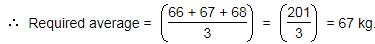QUESTION: 4

The average monthly income of P and Q is Rs. 5050. The average monthly income of Q and R is Rs. 6250 and the average monthly income of P and R is Rs. 5200. The monthly income of P is:

Solution:

Let P, Q and R represent their respective monthly incomes. Then, we have:

P + Q = (5050 x 2) = 10100 .... (i)

Q + R = (6250 x 2) = 12500 .... (ii)

P + R = (5200 x 2) = 10400 .... (iii)

Adding (i), (ii) and (iii), we get:  2(P + Q + R) = 33000  or   P + Q + R = 16500 .... (iv)

Subtracting (ii) from (iv), we get P = 4000.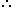P's monthly income = Rs. 4000.

QUESTION: 5

A grocer has a sale of Rs. 6435, Rs. 6927, Rs. 6855, Rs. 7230 and Rs. 6562 for 5 consecutive months. How much sale must he have in the sixth month so that he gets an average sale of Rs. 6500?

Solution:

Total sale for 5 months = Rs. (6435 + 6927 + 6855 + 7230 + 6562) = Rs. 34009.Required sale = Rs. [ (6500 x 6) - 34009 ]

= Rs. (39000 - 34009)

= Rs. 4991.

QUESTION: 6

The captain of a cricket team of 11 members is 26 years old and the wicket keeper is 3 years older. If the ages of these two are excluded, the average age of the remaining players is one year less than the average age of the whole team. Find out the average age of the team.

Solution:

Let the average age of the whole team by x years.11x - (26 + 29) = 9(x -1)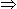11x - 9x = 462x = 46x = 23.

So, average age of the team is 23 years.

QUESTION: 7

A student's marks were wrongly entered as 83 instead of 63. Due to that, the average marks for the class got increased by 1/2. What is the number of students in the class?

Solution:

Let there be x students in the class.

Total increase in marks =( x * 1/2)

= x/2x/2 = (83 - 63)x/2 = 20x= 40

QUESTION: 8

The average weight of 16 boys in a class is 50.25 kg and that of the remaining 8 boys is 45.15 kg. Find the average weights of all the boys in the class.

Solution: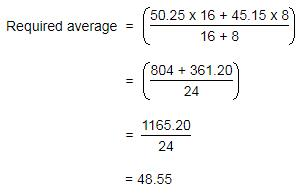QUESTION: 9

The average of 20 numbers is zero. Of them, how many of them may be greater than zero, at the most?

Solution:

Average of 20 numbers = 0.Sum of 20 numbers (0 x 20) = 0.

It is quite possible that 19 of these numbers may be positive and if their sum is a then 20th number is (-a).

QUESTION: 10

A library has an average of 510 visitors on Sundays and 240 on other days. What is the average number of visitors per day in a month of 30 days beginning with a Sunday?

Solution: